We’d like to understand how you use our websites in order to improve them. Register your interest.

# Data dependence results of new multi-step and S-iterative schemes for contractive-like operators

## Abstract

In this paper, we prove that the convergence of a new iteration and S-iteration can be used to approximate the fixed points of contractive-like operators. We also prove some data dependence results for these new iteration and S-iteration schemes for contractive-like operators. Our results extend and improve some known results in the literature.

MSC:47H10.

## 1 Introduction

Contractive mappings and iteration procedures are some of the main tools in the study of fixed point theory. There are many contractive mappings and iteration schemes that have been introduced and developed by several authors to serve various purposes in the literature of this highly active research area, viz.,  among others.

Whether an iteration method used in any investigation converges to a fixed point of a contractive type mapping corresponding to a particular iteration process is of utmost importance. Therefore it is natural to see many works related to the convergence of iteration methods such as .

Fixed point theory is concerned with investigating a wide variety of issues such as the existence (and uniqueness) of fixed points, the construction of fixed points, etc. One of these themes is data dependency of fixed points. Data dependency of fixed points has been the subject of research in fixed point theory for some time now, and data dependence research is an important theme in its own right.

Several authors who have made contributions to the study of data dependence of fixed points are Rus and Muresan , Rus et al. [24, 25], Berinde , Espínola and Petruşel , Markin , Chifu and Petruşel , Olantiwo [30, 31], Şoltuz [32, 33], Şoltuz and Grosan , Chugh and Kumar  and the references therein.

This paper is organized as follows. In Section 1 we present a brief survey of some known contractive mappings and iterative schemes and collect some preliminaries that will be used in the proofs of our main results. In Section 2 we show that the convergence of a new multi-step iteration, which is a special case of the Jungck multistep-SP iterative process defined in , and S-iteration (due to Agarwal et al.) can be used to approximate the fixed points of contractive-like operators. Motivated by the works of Şoltuz [32, 33], Şoltuz and Grosan , and Chugh and Kumar , we prove two data dependence results for the new multi-step iteration and S-iteration schemes by employing contractive-like operators.

As a background of our exposition, we now mention some contractive mappings and iteration schemes.

In  Zamfirescu established an important generalization of the Banach fixed point theorem using the following contractive condition. For a mapping $T:E→E$, there exist real numbers a, b, c satisfying $0, $0 such that, for each pair $x,y∈X$, at least one of the following is true:

${ ( z 1 ) ∥ T x − T y ∥ ≤ a ∥ x − y ∥ , ( z 2 ) ∥ T x − T y ∥ ≤ b ( ∥ x − T x ∥ + ∥ y − T y ∥ ) , ( z 3 ) ∥ T x − T y ∥ ≤ c ( ∥ x − T y ∥ + ∥ y − T x ∥ ) .$
(1.1)

A mapping T satisfying the contractive conditions (z1), (z2) and (z3) in (1.1) is called a Zamfirescu operator. An operator satisfying condition (z2) is called a Kannan operator, while the mapping satisfying condition (z3) is called a Chatterjea operator. As shown in , the contractive condition (1.1) leads to

(1.2)

for all $x,y∈E$, where $δ:=max{a, b 1 − b , c 1 − c }$, $δ∈[0,1)$, and it was shown that this class of operators is wider than the class of Zamfirescu operators. Any mapping satisfying condition (b1) or (b2) is called a quasi-contractive operator.

Extending the above definition, Osilike and Udomene  considered operators T for which there exist real numbers $L≥0$ and $δ∈[0,1)$ such that for all $x,y∈E$,

$∥Tx−Ty∥≤δ∥x−y∥+L∥x−Tx∥.$
(1.3)

Imoru and Olantiwo  gave a more general definition: An operator T is called a contractive-like operator if there exists a constant $δ∈[0,1)$ and a strictly increasing and continuous function $φ:[0,∞)→[0,∞)$, with $φ(0)=0$, such that for each $x,y∈E$,

$∥Tx−Ty∥≤δ∥x−y∥+φ ( ∥ x − T x ∥ ) .$
(1.4)

A map satisfying (1.4) need not have a fixed point, even if E is complete. For example, let $E=[0,∞)$ and define T by

$Tx={ 1.0 , 0 ≤ x ≤ 0.8 , 0.6 , 0.8 < x .$

WLOG, assume that $x. Then, for $0≤x or $0.8, $∥Tx−Ty∥=0$, and (1.4) is automatically satisfied.

If $0≤x≤0.8, then $∥Tx−Ty∥=0.4$.

Define φ by $φ(t)=Lt$ for any $L≥2$. Then φ is increasing, continuous, and $φ(0)=0$. Also, $∥x−Tx∥=1−x$ so that $φ(∥x−Tx∥)=L(1−x)≥0.2L≥0.4$.

Therefore

$0.4=∥Tx−Ty∥≤L∥x−Tx∥≤δ∥x−y∥+L∥x−Tx∥$

for any $0≤δ<1$, and (1.4) is satisfied for $0≤x≤0.8. But T has no fixed point.

However, using (1.4) it is obvious that if T has a fixed point, then it is unique.

From now on, we demand that denotes the set of all nonnegative integers. Let X be a Banach space, let $E⊂X$ be a nonempty closed, convex subset of X, and let T be a self-map on E. Define $F T :={p∈X:p=Tp}$ to be the set of fixed points of T. Let ${ α n } n = 0 ∞$, ${ β n } n = 0 ∞$, ${ γ n } n = 0 ∞$ and ${ β n i } n = 0 ∞$, $i= 1 , k − 2 ¯$, $k≥2$ be real sequences in $[0,1)$ satisfying certain conditions.

In  Rhoades and Şoltuz introduced a multi-step iterative procedure given by

${ x 0 ∈ E , y n k − 1 = ( 1 − β n k − 1 ) x n + β n k − 1 T x n , k ≥ 2 , y n i = ( 1 − β n i ) x n + β n i T y n i + 1 , i = 1 , k − 2 ¯ , x n + 1 = ( 1 − α n ) x n + α n T y n 1 , n ∈ N .$
(1.5)

The sequence ${ x n } n = 0 ∞$ defined by

${ x 0 ∈ E , x n + 1 = ( 1 − α n ) T x n + α n T y n , y n = ( 1 − β n ) x n + β n T x n , n ∈ N ,$
(1.6)

is known as the S-iteration process (see [12, 17, 39]).

Thianwan  defined a two-step iteration ${ x n } n = 0 ∞$ by

${ x 0 ∈ E , x n + 1 = ( 1 − α n ) y n + α n T y n , y n = ( 1 − β n ) x n + β n T x n , n ∈ N .$
(1.7)

Recently Phuengrattana and Suantai  introduced an SP iteration method defined by

${ x 0 ∈ E , x n + 1 = ( 1 − α n ) y n + α n T y n , y n = ( 1 − β n ) z n + β n T z n , z n = ( 1 − γ n ) x n + γ n T x n , n ∈ N .$
(1.8)

We shall employ the following iterative process. For an arbitrary fixed order $k≥2$,

${ x 0 ∈ E , x n + 1 = ( 1 − α n ) y n 1 + α n T y n 1 , y n 1 = ( 1 − β n 1 ) y n 2 + β n 1 T y n 2 , y n 2 = ( 1 − β n 2 ) y n 3 + β n 2 T y n 3 , ⋯ , y n k − 2 = ( 1 − β n k − 2 ) y n k − 1 + β n k − 2 T y n k − 1 , y n k − 1 = ( 1 − β n k − 1 ) x n + β n k − 1 T x n , n ∈ N ,$
(1.9)

or, in short,

${ x 0 ∈ E , x n + 1 = ( 1 − α n ) y n 1 + α n T y n 1 , y n i = ( 1 − β n i ) y n i + 1 + β n i T y n i + 1 , i = 1 , k − 2 ¯ , y n k − 1 = ( 1 − β n k − 1 ) x n + β n k − 1 T x n , n ∈ N ,$
(1.10)

where

${ α n } n = 0 ∞ ⊂[0,1), ∑ n = 0 ∞ α n =∞,$
(1.11)

and

${ β n i } n = 0 ∞ ⊂[0,1),i= 1 , k − 1 ¯ .$
(1.12)

Remark 1 If each $γ n =0$, then SP iteration (1.8) reduces to two-step iteration (1.7). By taking $k=3$ and $k=2$ in (1.10), we obtain iterations (1.8) and (1.7), respectively.

We shall need the following definition and lemma in the sequel.

Definition 1 

Let $T, T ˜ :X→X$ be two operators. We say that $T ˜$ is an approximate operator for T if, for some $ε>0$, we have

$∥Tx− T ˜ x∥≤ε$

for all $x∈X$.

Lemma 1 

Let ${ a n } n = 0 ∞$ be a nonnegative sequence for which one assumes that there exists an $n 0 ∈N$ such that for all $n≥ n 0$,

$a n + 1 ≤(1− μ n ) a n + μ n η n$

is satisfied, where $μ n ∈(0,1)$ for all $n∈N$, $∑ n = 0 ∞ μ n =∞$ and $η n ≥0$, $∀n∈N$. Then the following holds:

$0≤ lim n → ∞ sup a n ≤ lim n → ∞ sup η n .$

## 2 Main results

For simplicity we use the following notation throughout this section.

For any iterative process, ${ x n } n = 0 ∞$ and ${ u n } n = 0 ∞$ denote iterative sequences associated to T and $T ˜$, respectively.

Theorem 1 Let $T:E→E$ be a map satisfying (1.4) with $F T ≠∅$, and let ${ x n } n = 0 ∞$ be a sequence defined by (1.10), then the sequence ${ x n } n = 0 ∞$ converges to the unique fixed point of T.

Proof The proof can be easily obtained by using the argument in the proof of (, Theorem 3.1). □

This result allows us to give the next theorem.

Theorem 2 Let $T:E→E$ be a map satisfying (1.4) with $F T ≠∅$, and let $T ˜$ be an approximate operator of T as in Definition  1. Let ${ x n } n = 0 ∞$, ${ u n } n = 0 ∞$ be two iterative sequences defined by (1.10) with real sequences ${ α n } n = 0 ∞ , { β n i } n = 0 ∞ ⊂[0,1)$ satisfying (i) $0≤ β n i < α n ≤1$, $i= 1 , k − 1 ¯$, (ii) $∑ α n =∞$. If $p=Tp$ and $q= T ˜ q$, then we have

$∥p−q∥≤ k ε 1 − δ .$

Proof For given $x 0 ∈E$ and $u 0 ∈E$, we consider the following multi-step iteration for T and $T ˜$:

${ x 0 ∈ E , x n + 1 = ( 1 − α n ) y n 1 + α n T y n 1 , y n i = ( 1 − β n i ) y n i + 1 + β n i T y n i + 1 , i = 1 , k − 2 ¯ , y n k − 1 = ( 1 − β n k − 1 ) x n + β n k − 1 T x n , k ≥ 2 , n ∈ N ,$
(2.1)

and

${ u 0 ∈ E , u n + 1 = ( 1 − α n ) v n 1 + α n T ˜ v n 1 , v n i = ( 1 − β n i ) v n i + 1 + β n i T ˜ v n i + 1 , i = 1 , k − 2 ¯ , v n k − 1 = ( 1 − β n k − 1 ) u n + β n k − 1 T ˜ u n , k ≥ 2 , n ∈ N .$
(2.2)

Thus, from (1.4), (2.1) and (2.2), we have the following inequalities.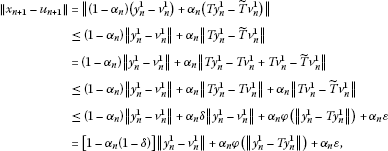(2.3)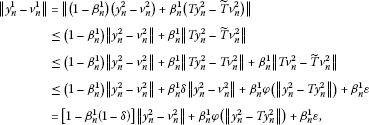(2.4)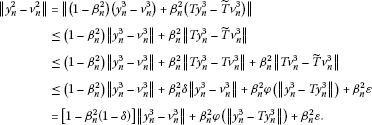(2.5)

Combining (2.3), (2.4) and (2.5), we obtain

$∥ x n + 1 − u n + 1 ∥ ≤ [ 1 − α n ( 1 − δ ) ] [ 1 − β n 1 ( 1 − δ ) ] [ 1 − β n 2 ( 1 − δ ) ] ∥ y n 3 − v n 3 ∥ + [ 1 − α n ( 1 − δ ) ] [ 1 − β n 1 ( 1 − δ ) ] β n 2 φ ( ∥ y n 3 − T y n 3 ∥ ) + [ 1 − α n ( 1 − δ ) ] [ 1 − β n 1 ( 1 − δ ) ] β n 2 ε + [ 1 − α n ( 1 − δ ) ] β n 1 φ ( ∥ y n 2 − T y n 2 ∥ ) + [ 1 − α n ( 1 − δ ) ] β n 1 ε + α n φ ( ∥ y n 1 − T y n 1 ∥ ) + α n ε .$
(2.6)

Thus, by induction, we get

$∥ x n + 1 − u n + 1 ∥ ≤ [ 1 − α n ( 1 − δ ) ] [ 1 − β n 1 ( 1 − δ ) ] ⋯ [ 1 − β n k − 2 ( 1 − δ ) ] ∥ y n k − 1 − v n k − 1 ∥ + [ 1 − α n ( 1 − δ ) ] [ 1 − β n 1 ( 1 − δ ) ] ⋯ [ 1 − β n k − 3 ( 1 − δ ) ] β n k − 2 φ ( ∥ y n k − 1 − T y n k − 1 ∥ ) + ⋯ + [ 1 − α n ( 1 − δ ) ] β n 1 φ ( ∥ y n 2 − T y n 2 ∥ ) + α n φ ( ∥ y n 1 − T y n 1 ∥ ) + [ 1 − α n ( 1 − δ ) ] [ 1 − β n 1 ( 1 − δ ) ] ⋯ [ 1 − β n k − 3 ( 1 − δ ) ] β n k − 2 ε + ⋯ + [ 1 − α n ( 1 − δ ) ] β n 1 ε + α n ε .$
(2.7)

Again, using (1.4), (2.1) and (2.2), we get

$∥ y n k − 1 − v n k − 1 ∥ = ∥ ( 1 − β n k − 1 ) ( x n − u n ) + β n k − 1 ( T x n − T ˜ u n ) ∥ ≤ ( 1 − β n k − 1 ) ∥ x n − u n ∥ + β n k − 1 ∥ T x n − T ˜ u n ∥ ≤ ( 1 − β n k − 1 ) ∥ x n − u n ∥ + β n k − 1 ∥ T x n − T u n ∥ + β n k − 1 ∥ T u n − T ˜ u n ∥ ≤ [ 1 − β n k − 1 ( 1 − δ ) ] ∥ x n − u n ∥ + β n k − 1 φ ( ∥ x n − T x n ∥ ) + β n k − 1 ε .$
(2.8)

Substituting (2.8) in (2.7), we have

$∥ x n + 1 − u n + 1 ∥ ≤ [ 1 − α n ( 1 − δ ) ] [ 1 − β n 1 ( 1 − δ ) ] ⋯ [ 1 − β n k − 1 ( 1 − δ ) ] ∥ x n − u n ∥ + [ 1 − α n ( 1 − δ ) ] [ 1 − β n 1 ( 1 − δ ) ] ⋯ [ 1 − β n k − 2 ( 1 − δ ) ] β n k − 1 φ ( ∥ x n − T x n ∥ ) + [ 1 − α n ( 1 − δ ) ] [ 1 − β n 1 ( 1 − δ ) ] ⋯ [ 1 − β n k − 3 ( 1 − δ ) ] β n k − 2 φ ( ∥ y n k − 1 − T y n k − 1 ∥ ) + ⋯ + [ 1 − α n ( 1 − δ ) ] β n 1 φ ( ∥ y n 2 − T y n 2 ∥ ) + α n φ ( ∥ y n 1 − T y n 1 ∥ ) + [ 1 − α n ( 1 − δ ) ] [ 1 − β n 1 ( 1 − δ ) ] ⋯ [ 1 − β n k − 2 ( 1 − δ ) ] β n k − 1 ε + ⋯ + [ 1 − α n ( 1 − δ ) ] β n 1 ε + α n ε .$
(2.9)

Since $δ∈[0,1)$ and ${ α n } n = 0 ∞ , { β n i } n = 0 ∞ ⊂[0,1)$, for $i= 1 , k − 1 ¯$, we have

$[ 1 − α n ( 1 − δ ) ] [ 1 − β n 1 ( 1 − δ ) ] ⋯ [ 1 − β n i ( 1 − δ ) ] ≤ [ 1 − α n ( 1 − δ ) ] .$
(2.10)

From inequality (2.10) and assumption (i) in (2.9), it follows

$∥ x n + 1 − u n + 1 ∥ ≤ [ 1 − α n ( 1 − δ ) ] ∥ x n − u n ∥ + α n φ ( ∥ x n − T x n ∥ ) + α n φ ( ∥ y n k − 1 − T y n k − 1 ∥ ) + ⋯ + α n φ ( ∥ y n 2 − T y n 2 ∥ ) + α n φ ( ∥ y n 1 − T y n 1 ∥ ) + α n ε + α n ε + ⋯ + α n ε + α n ε = [ 1 − α n ( 1 − δ ) ] ∥ x n − u n ∥ + α n ( 1 − δ ) { φ ( ∥ x n − T x n ∥ ) + φ ( ∥ y n k − 1 − T y n k − 1 ∥ ) 1 − δ + ⋯ + φ ( ∥ y n 1 − T y n 1 ∥ ) + k ε 1 − δ } .$
(2.11)

Define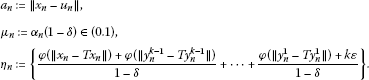From Theorem 1 it follows that $lim n → ∞ ∥ x n −p∥=0$. Since T satisfies condition (1.4) and $Tp=p∈ F T$,

(2.12)

Since $β n i ∈[0,1)$, $∀n∈N$, $i= 1 , k − 1 ¯$, using (1.4) and (1.10), we have

(2.13)

It is easy to see from (2.13) that this result is also valid for $∥T y n 2 − y n 2 ∥,…,∥T y n k − 1 − y n k − 1 ∥$.

Since φ is continuous, we have

$lim n → ∞ φ ( ∥ x n − T x n ∥ ) = lim n → ∞ φ ( ∥ y n 1 − T y n 1 ∥ ) =⋯= lim n → ∞ φ ( ∥ y n k − 1 − T y n k − 1 ∥ ) =0.$
(2.14)

Hence an application of Lemma 1 to (2.11) leads to

$∥p−q∥≤ k ε 1 − δ .$
(2.15)

□

As shown by Hussain et al. (, Theorem 8), in an arbitrary Banach space X, the S-iteration ${ x n } n = 0 ∞$ given by (1.6) converges to the fixed point of T, where $T:E→E$ is a mapping satisfying condition (1.3).

Theorem 3 Let $T:E→E$ be a map satisfying (1.4) with $F T ≠∅$, and let ${ x n } n = 0 ∞$ be defined by (1.6) with real sequences ${ β n } n = 0 ∞ , { α n } n = 0 ∞ ⊂[0,1)$ satisfying $∑ n = 0 ∞ α n =∞$. Then the sequence ${ x n } n = 0 ∞$ converges to the unique fixed point of T.

Proof The argument is similar to the proof of Theorem 8 of , and is thus omitted. □

We now prove the result on data dependence for the S-iterative procedure by utilizing Theorem 3.

Theorem 4 Let T, $T ˜$ be two operators as in Theorem  2. Let ${ x n } n = 0 ∞$, ${ u n } n = 0 ∞$ be S-iterations defined by (1.6) with real sequences ${ β n } n = 0 ∞ , { α n } n = 0 ∞ ⊂[0,1)$ satisfying (i) $1 2 ≤ α n$, $∀n∈N$, and (ii) $∑ n = 0 ∞ α n =∞$. If $p=Tp$ and $q= T ˜ q$, then we have

$∥p−q∥≤ 3 ε 1 − δ .$

Proof For a given $x 0 ∈E$ and $u 0 ∈E$, we consider the following iteration for T and $T ˜$:

${ x 0 ∈ E , x n + 1 = ( 1 − α n ) T x n + α n T y n , y n = ( 1 − β n ) x n + β n T x n , n ∈ N$
(2.16)

and

${ u 0 ∈ E , u n + 1 = ( 1 − α n ) T ˜ u n + α n T ˜ v n , v n = ( 1 − β n ) u n + β n T ˜ u n , n ∈ N .$
(2.17)

Using (1.4), (2.16) and (2.17), we obtain the following estimates: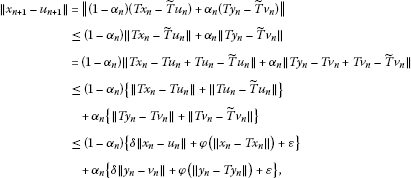(2.18)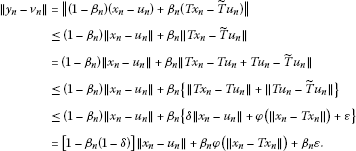(2.19)

Combining (2.18) and (2.19), we get

$∥ x n + 1 − u n + 1 ∥ ≤ { ( 1 − α n ) δ + α n δ [ 1 − β n ( 1 − δ ) ] } ∥ x n − u n ∥ + { 1 − α n + α n δ β n } φ ( ∥ x n − T x n ∥ ) + α n φ ( ∥ y n − T y n ∥ ) + α n δ β n ε + ( 1 − α n ) ε + α n ε .$
(2.20)

For ${ α n } n = 0 ∞ , { β n } n = 0 ∞ ⊂[0,1)$ and $δ∈[0,1)$,

$(1− α n )δ<1− α n ,1− β n (1−δ)<1, α n δ β n < α n .$
(2.21)

It follows from assumption (i) that

$1− α n < α n ,∀n∈N.$
(2.22)

Therefore, combining (2.22) and (2.21) to (2.20) gives

$∥ x n + 1 − u n + 1 ∥ ≤ [ 1 − α n ( 1 − δ ) ] ∥ x n − u n ∥ + 2 α n φ ( ∥ x n − T x n ∥ ) + α n φ ( ∥ y n − T y n ∥ ) + α n ε + α n ε + α n ε ,$
(2.23)

or, equivalently,

$∥ x n + 1 − u n + 1 ∥ ≤ [ 1 − α n ( 1 − δ ) ] ∥ x n − u n ∥ + α n ( 1 − δ ) { 2 φ ( ∥ x n − T x n ∥ ) + φ ( ∥ y n − T y n ∥ ) + 3 ε } 1 − δ .$
(2.24)

Now define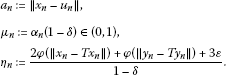From Theorem 3, we have $lim n → ∞ ∥ x n −p∥=0$. Since T satisfies condition (1.4), and $Tp=p∈ F T$, using an argument similar to that in the proof of Theorem 2,

$lim n → ∞ ∥ x n −T x n ∥= lim n → ∞ ∥ y n −T y n ∥=0.$
(2.25)

Using the fact that φ is continuous, we have

$lim n → ∞ φ ( ∥ x n − T x n ∥ ) = lim n → ∞ φ ( ∥ y n − T y n ∥ ) =0.$
(2.26)

An application of Lemma 1 to (2.24) leads to

$∥p−q∥≤ 3 ε 1 − δ .$
(2.27)

□

## 3 Conclusion

Since the iterative schemes (1.7) and (1.8) are special cases of the iterative process (1.10), Theorem 1 generalizes Theorem 2.1 of  and Theorem 2.1 of . By taking $k=3$ and $k=2$ in Theorem 2, data dependence results for the iterative schemes (1.8) and (1.7) can be easily obtained. For $k=3$, Theorem 2 reduces to Theorem 3.2 of . Since condition (1.4) is more general than condition (1.3), Theorem 3 generalizes Theorem 8 of .

## References

1. 1.

Rhoades BE: A comparison of various definitions of contractive mappings. Trans. Am. Math. Soc. 1977, 226: 257–290.

2. 2.

Mann WR: Mean value methods in iterations. Proc. Am. Math. Soc. 1953, 4: 506–510. 10.1090/S0002-9939-1953-0054846-3

3. 3.

Ishikawa S: Fixed points by a new iteration method. Proc. Am. Math. Soc. 1974, 44: 147–150. 10.1090/S0002-9939-1974-0336469-5

4. 4.

Noor MA: New approximation schemes for general variational inequalities. J. Math. Anal. Appl. 2000, 251: 217–229. 10.1006/jmaa.2000.7042

5. 5.

Rhoades BE, Şoltuz SM: The equivalence between Mann-Ishikawa iterations and multistep iteration. Nonlinear Anal. 2004, 58: 219–228. 10.1016/j.na.2003.11.013

6. 6.

Thianwan S: Common fixed points of new iterations for two asymptotically nonexpansive nonself mappings in a Banach space. J. Comput. Appl. Math. 2008. doi:10.1016/j.cam.2008.05.051

7. 7.

Phuengrattana W, Suantai S: On the rate of convergence of Mann, Ishikawa, Noor and SP iterations for continuous functions on an arbitrary interval. J. Comput. Appl. Math. 2011, 235: 3006–3014. 10.1016/j.cam.2010.12.022

8. 8.

Glowinski R, Le Tallec P: Augmented Langrangian and Operator Splitting Methods in Nonlinear Mechanics. SIAM, Philadelphia; 1989.

9. 9.

Xu B, Noor MA: Ishikawa and Mann iteration process with errors for nonlinear strongly accretive operator equations. J. Math. Anal. Appl. 1998, 224: 91–101. 10.1006/jmaa.1998.5987

10. 10.

Takahashi W: Iterative methods for approximation of fixed points and their applications. J. Oper. Res. Soc. Jpn. 2000, 43: 87–108.

11. 11.

Das G, Debata JP: Fixed points of quasi-nonexpansive mappings. Indian J. Pure Appl. Math. 1986, 17: 1263–1269.

12. 12.

Agarwal RP, O’Regan D, Sahu DR: Iterative construction of fixed points of nearly asymptotically nonexpansive mappings. J. Nonlinear Convex Anal. 2007, 8: 61–79.

13. 13.

Berinde V: On the convergence of the Ishikawa iteration in the class of quasi contractive operators. Acta Math. Univ. Comen. 2004, 73: 119–126.

14. 14.

Chidume CE, Chidume CO: Convergence theorem for fixed points of uniformly continuous generalized phihemicontractive mappings. J. Math. Anal. Appl. 2005, 303: 545–554. 10.1016/j.jmaa.2004.08.060

15. 15.

Chidume CE, Chidume CO: Iterative approximation of fixed points of nonexpansive mappings. J. Math. Anal. Appl. 2006, 318: 288–295. 10.1016/j.jmaa.2005.05.023

16. 16.

Suantai S: Weak and strong convergence criteria of Noor iterations for asymptotically nonexpansive mappings. J. Math. Anal. Appl. 2005, 311: 506–517. 10.1016/j.jmaa.2005.03.002

17. 17.

Sahu DR: Applications of the S-iteration process to constrained minimization problems and split feasibility problems. Fixed Point Theory Appl. 2011, 12: 187–204.

18. 18.

Yıldırım İ, Özdemir M, Kızıltunç H: On the convergence of a new two-step iteration in the class of quasi-contractive operators. Int. J. Math. Anal. 2009, 3: 1881–1892.

19. 19.

Chugh R, Kumar V: Strong convergence of SP iterative scheme for quasi-contractive operators in Banach spaces. Int. J. Comput. Appl. 2011, 31: 21–27.

20. 20.

Osilike MO, Udomene A: Short proofs of stability results for fixed point iteration procedures for a class of contractive-type mappings. Indian J. Pure Appl. Math. 1999, 30: 1229–1234.

21. 21.

Rafiq A: On the convergence of the three step iteration process in the class of quasi-contractive operators. Acta Math. Acad. Paedagog. Nyházi. 2006, 22: 305–309.

22. 22.

Hussain N, Rafiq A, Damjanović B, Lazović R: On rate of convergence of various iterative schemes. Fixed Point Theory Appl. 2011., 2011: Article ID 45. doi:10.1186/1687–1812–2011–45

23. 23.

Rus IA, Muresan S: Data dependence of the fixed points set of weakly Picard operators. Stud. Univ. Babeş-Bolyai, Math. 1998, 43: 79–83.

24. 24.

Rus IA, Petruşel A, Sîntamarian A: Data dependence of the fixed points set of multivalued weakly Picard operators. Stud. Univ. Babeş-Bolyai, Math. 2001, 46: 111–121.

25. 25.

Rus IA, Petruşel A, Sîntamarian A: Data dependence of the fixed point set of some multivalued weakly Picard operators. Nonlinear Anal., Theory Methods Appl. 2003, 52: 1947–1959. 10.1016/S0362-546X(02)00288-2

26. 26.

Berinde V: On the approximation of fixed points of weak contractive mappings. Carpath. J. Math. 2003, 19: 7–22.

27. 27.

Espínola R, Petruşel A: Existence and data dependence of fixed points for multivalued operators on gauge spaces. J. Math. Anal. Appl. 2005, 309: 420–432. 10.1016/j.jmaa.2004.07.006

28. 28.

Markin JT: Continuous dependence of fixed point sets. Proc. Am. Math. Soc. 1973, 38: 545–547. 10.1090/S0002-9939-1973-0313897-4

29. 29.

Chifu C, Petruşel G: Existence and data dependence of fixed points and strict fixed points for contractive-type multivalued operators. Fixed Point Theory Appl. 2007. doi:10.1155/2007/34248

30. 30.

Olatinwo MO: Some results on the continuous dependence of the fixed points in normed linear space. Fixed Point Theory Appl. 2009, 10: 151–157.

31. 31.

Olatinwo MO:On the continuous dependence of the fixed points for $(φ,ψ)$-contractive-type operators. Kragujev. J. Math. 2010, 34: 91–102.

32. 32.

Şoltuz SM: Data dependence for Mann iteration. Octogon Math. Mag. 2001, 9: 825–828.

33. 33.

Şoltuz SM: Data dependence for Ishikawa iteration. Lect. Mat. 2004, 25: 149–155.

34. 34.

Şoltuz SM, Grosan T: Data dependence for Ishikawa iteration when dealing with contractive like operators. Fixed Point Theory Appl. 2008., 2008: Article ID 242916. doi:10.1155/2008/242916

35. 35.

Chugh R, Kumar V: Data dependence of Noor and SP iterative schemes when dealing with quasi-contractive operators. Int. J. Comput. Appl. 2011, 40: 41–46.

36. 36.

Akewe H: Strong convergence and stability of Jungck-multistep-SP iteration for generalized contractive-like inequality operators. Adv. Nat. Sci. 2012, 5: 21–27.

37. 37.

Zamfirescu T: Fix point theorems in metric spaces. Arch. Math. 1972, 23: 292–298. 10.1007/BF01304884

38. 38.

Imoru CO, Olantiwo MO: On the stability of Picard and Mann iteration processes. Carpath. J. Math. 2003, 19: 155–160.

39. 39.

Agarwal RP, O’Regan D, Sahu DR: Fixed Point Theory for Lipschitzian Type-Mappings with Applications. Springer, New York; 2009.

40. 40.

Berinde V: Iterative Approximation of Fixed Points. Springer, Berlin; 2007.

## Acknowledgements

The first two authors would like to thank Yıldız Technical University Scientific Research Projects Coordination Unit under project number BAPK 2012-07-03-DOP02 for financial support during the preparation of this manuscript.

## Author information

Authors

### Corresponding author

Correspondence to Vatan Karakaya.

### Competing interests

The authors declare that they have no competing interests.

### Authors’ contributions

All authors contributed equally and significantly in writing this article. All authors read and approved the final manuscript.

## Rights and permissions

Reprints and Permissions

Gürsoy, F., Karakaya, V. & Rhoades, B.E. Data dependence results of new multi-step and S-iterative schemes for contractive-like operators. Fixed Point Theory Appl 2013, 76 (2013). https://doi.org/10.1186/1687-1812-2013-76